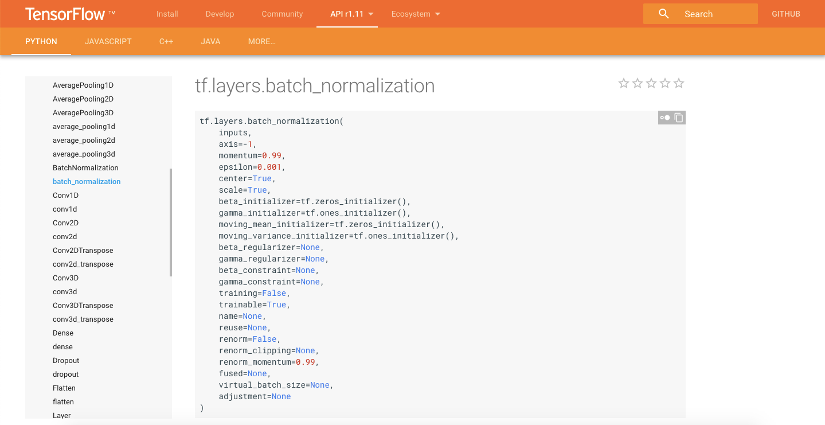# Using TensorFlow’s Batch Normalization Correctly

· Timo Denk

Update: This guide applies to TF1. For TF2, use tf.keras.layers.BatchNormalization layer.

The TensorFlow library’s layers API contains a function for batch normalization: tf.layers.batch_normalization. It is supposedly as easy to use as all the other tf.layers functions, however, it has some pitfalls. This post explains how to use tf.layers.batch_normalization correctly. It does not delve into what batch normalization is, which can be looked up in the paper “Batch Normalization: Accelerating Deep Network Training by Reducing Internal Covariate Shift” by Ioeffe and Szegedy (2015).

Summary

• Use the training parameter of the batch_normalization function.
• Update the moving averages by evaluating the ops manually or by adding them as a control dependency.
• The final code can be found in this Jupyter notebook.

We start off by defining a simple computational graph. The input is a placeholder which takes a batch of scalar values.

x = tf.placeholder(tf.float32, [None, 1], 'x')


The input $x$ is fed into a batch normalization layer, yielding the graph’s output $y$:

y = tf.layers.batch_normalization(x)


With this setup we have got some basic batch normalization set up. We can create a session and feed a sample vector, here $x=\begin{bmatrix}-10 & 0 & 10\end{bmatrix}$.

sess = tf.Session()
init = tf.global_variables_initializer()
sess.run(init)
y_out = sess.run(y, feed_dict={x: [[-10], , ]})
sess.close()


Surprisingly, the value of y_out is not normalized at all. In fact it is something like $y=\begin{bmatrix}-9.995004 & 0 & 9.995004\end{bmatrix}$.

The batch normalization layer does not normalize based on the current batch if its training parameter is not set to true. Heading back to the definition of $y$, we can alter the method call a bit:

y = tf.layers.batch_normalization(x, training=True)


After making this change, the output for $y$ looks much more normalized. It is something like $y=\begin{bmatrix}-1.2247357 & 0 & 1.2247357\end{bmatrix}$.

With this setup, the batch normalization layer looks at the current batch and normalized it depending on its value. That might not be desired, at any time: Consider a case where there is only one sample in the batch, e.g. $x=\begin{bmatrix}-10\end{bmatrix}$. In this setup, the output would be $y=\begin{bmatrix}0\end{bmatrix}$ because that way the batch is normalized to zero-mean. Therefore it is common to store so called moving averages of mean and variance, to be able to choose the normalization factors $\beta$ and $\gamma$ properly. That way smaller batches can be normalized with the same parameters as batches before.

In order to update the two moving average variables (mean and variance), which the tf.layers.batch_normalization function call creates automatically, two operations must be evaluated while feeding a batch through the layer. The operations can be found in the collection tf.GraphKeys.UPDATE_OPS. In the example above, tf.get_collection(tf.GraphKeys.UPDATE_OPS) yields

[<tf.Operation 'batch_normalization/AssignMovingAvg' type=AssignSub>,
<tf.Operation 'batch_normalization/AssignMovingAvg_1' type=AssignSub>]


If we change the setup and evaluate the update operations alongside with the forward pass

update_ops = tf.get_collection(tf.GraphKeys.UPDATE_OPS)
y_1 = sess.run([y, update_ops], feed_dict={x: [[-10], , ]})
y_2 = sess.run(y, feed_dict={x: [[-10]]})


the values for $y_1$ and $y_2$ remain the same. That is because the moving averages are only being used, if the training parameter is set to False. We can control it with a placeholder (here a placeholder with a default value)

is_training = tf.placeholder_with_default(False, (), 'is_training')
y = tf.layers.batch_normalization(x, training=is_training)


and set it to True when feeding the larger batch (and False for the smaller batch; strictly not necessary because it is the placeholder’s default value anyways):

y_1 = sess.run([y, update_ops], feed_dict={x: [[-10], , ], is_training: True})
y_2 = sess.run(y, feed_dict={x: [[-10]], is_training: False})


The output we get is

• $y_1=\begin{bmatrix}-1.2247357 & 0 & 1.2247357\end{bmatrix}$: Normalized as desired, moving averages were updated based on this normalization.
• $y_2=\begin{bmatrix}-7.766966\end{bmatrix}$: Kind of weird. It’s neither $0$, which it was without moving averages, nor $-1.2247357$, which it should be if it was normalized with the same factors as the $x_1$ batch.

The reason for the wrong normalization of the small batch is that the moving averages update slowly. If we were to feed the larger batch multiple times, the second batch would be properly normalized:

for _ in range(1000):
y_1 = sess.run([y, update_ops], feed_dict={x: [[-10], , ], is_training: True})
y_2 = sess.run(y, feed_dict={x: [[-10]], is_training: False})


Here, we feed the larger batch 1000 times and run the update operations every time. The result is $y_2=\begin{bmatrix}-1.224762\end{bmatrix}$ — the normalization based on moving averages works. By altering the momentum parameter of tf.layers.batch_normalization the pace of the average update can be adjusted.

Right now we have to call sess.run and pass the update_ops manually. It is more convenient to add them as a control dependency, such that TensorFlow always executes them if the Tensor y is being evaluated. The new graph definition looks like that:

x = tf.placeholder(tf.float32, [None, 1], 'x')
y = tf.layers.batch_normalization(x, training=is_training)

update_ops = tf.get_collection(tf.GraphKeys.UPDATE_OPS)
with tf.control_dependencies(update_ops):
y = tf.identity(y)


The forward passes are now much cleaner:

x_1 = [[-10], , ]
x_2 = [[-10]]
for _ in range(1000):
y_1 = sess.run(y, feed_dict={x: x_1, is_training: True})
y_2 = sess.run(y, feed_dict={x: x_2})


Typically, is_training should be set to True during training and False when performing inference.

The values stored by the batch normalization layer can be examined. In order to so, we retrieve their names from tf.all_variables() which outputs

[<tf.Variable 'batch_normalization/gamma:0' shape=(1,) dtype=float32_ref>,
<tf.Variable 'batch_normalization/beta:0' shape=(1,) dtype=float32_ref>,
<tf.Variable 'batch_normalization/moving_mean:0' shape=(1,) dtype=float32_ref>,
<tf.Variable 'batch_normalization/moving_variance:0' shape=(1,) dtype=float32_ref>]


in our toy example. The last two variables contain the moving averages of mean and variance of the past batches (for which the update ops were evaluated and training was set to True). The actual values can be queried as follows:

with tf.variable_scope("", reuse=tf.AUTO_REUSE):
out = sess.run([tf.get_variable('batch_normalization/moving_mean'),
tf.get_variable('batch_normalization/moving_variance')])
moving_average, moving_variance = out